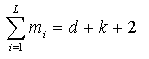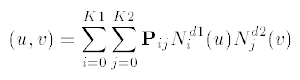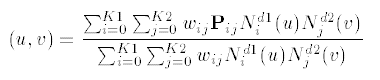# IFC 4.3.x development

## 8.9.3.7 IfcBSplineSurface

### 8.9.3.7.1 Semantic definitions at the entity

The IfcBSplineSurface is a general form of rational or polynomial parametric surface.

NOTE  Definition according to ISO/CD 10303-42:1992
A b_spline_surface is a general form of rational or polynomial parametric surface which is represented by control points, basis functions, and possibly, weights. As with the corresponding curve entity it has some special subtypes where some of the data can be derived.

1. The symbology used here is:
 K1 = upper_index_on_u_control_points K2 = upper_index_on_v_control_points Pij = control_points wij = weights d1 = u_degree d2 = v_degree

2. The control points are ordered as
P00, P01, P02, ......, PK1(K2-1), PK1K2
The weights, in the case of the rational subtype, are ordered similarly.

3. For each parameter, s = u or v, if k is the upper index on the control points and d is the degree for s, the knot array is an array of (k + d + 2) real numbers [s-d, ...., sk+1], such that for all indices j in [-d, k]; sjsj+1. This array is obtained from the appropriate u_knots or v_knots list by repeating each multiple knot according to the multiplicity.

Nid, the ith normalised B-spline basis function of degree d, is defined on the subset [si-d, ...., si+1] of this array.

4. Let L denote the number of distinct values amongst the knots in the knot list; L will be referred to as the ‘upper index on knots’. Let mj denote the multiplicity (i.e., number of repetitions) of the jth distinct knot value. Then:All knot multiplicities except the first and the last shall be in the range 1, ...., d; the first and last may have a maximum value of d+1. In evaluating the basis functions, a knot u of, e.g., multiplicity 3 is interpreted as a sequence u, u, u, in the knot array.

5. The surface form is used to identify specific quadric surface types (which shall have degree two), ruled surfaces and surfaces of revolution. As with the b-spline curve, the surface form is informational only and the spline data takes precedence.

6. The surface is to be interpreted as follows: In the polynomial case the surface is given by the equation:In the rational case the surface equation is:7. NOTE  Entity adapted from b_spline_surface defined in ISO10303-42.

HISTORY  New entity in IFC4.

### 8.9.3.7.1 Attributes

# Attribute Type Description
IfcRepresentationItem
LayerAssignment SET [0:1] OF IfcPresentationLayerAssignment FOR AssignedItemsAssignment of the representation item to a single or multiple layer(s). The LayerAssignments can override a LayerAssignments of the IfcRepresentation it is used within the list of Items.

> IFC2x3 CHANGE  The inverse attribute LayerAssignments has been added.

> IFC4 CHANGE  The inverse attribute LayerAssignment has been restricted to max 1. Upward compatibility for file based exchange is guaranteed.

StyledByItem SET [0:1] OF IfcStyledItem FOR Item Reference to the IfcStyledItem that provides presentation information to the representation, e.g. a curve style, including colour and thickness to a geometric curve.

> IFC2x3 CHANGE  The inverse attribute StyledByItem has been added.

IfcGeometricRepresentationItem
IfcSurface
Dim INVALID The space dimensionality of IfcSurface. It is always a three-dimensional geometric representation item.

> IFC4 CHANGE Derived attribute promoted from subtypes.

IfcBoundedSurface
IfcBSplineSurface
1 UDegree IfcInteger Algebraic degree of basis functions in u.
2 VDegree IfcInteger Algebraic degree of basis functions in v.
3 ControlPointsListLIST [2:?] OF LIST [2:?] OF IfcCartesianPoint This is a list of lists of control points.
4 SurfaceForm IfcBSplineSurfaceForm Indicator of special surface types.
5 UClosed IfcLogical Indication of whether the surface is closed in the u direction; this is for information only.
6 VClosed IfcLogical Indication of whether the surface is closed in the v direction; this is for information only.
7 SelfIntersect IfcLogical Flag to indicate whether, or not, surface is self-intersecting; this is for information only.
UUpper INVALID Upper index on control points in u direction.
VUpper INVALID Upper index on control points in v direction.
ControlPoints INVALID Array (two-dimensional) of control points defining surface geometry. This array is constructed from the control points list.

### 8.9.3.7.3 Formal representations

```ENTITY IfcBSplineSurface
ABSTRACT SUPERTYPE OF (ONEOF
(IfcBSplineSurfaceWithKnots))
SUBTYPE OF (IfcBoundedSurface);
UDegree : IfcInteger;
VDegree : IfcInteger;
ControlPointsList : LIST [2:?] OF LIST [2:?] OF IfcCartesianPoint;
SurfaceForm : IfcBSplineSurfaceForm;
UClosed : IfcLogical;
VClosed : IfcLogical;
SelfIntersect : IfcLogical;
DERIVE
UUpper : IfcInteger := SIZEOF(ControlPointsList) - 1;
VUpper : IfcInteger := SIZEOF(ControlPointsList) - 1;
ControlPoints : ARRAY [0:UUpper] OF ARRAY [0:VUpper] OF IfcCartesianPoint := IfcMakeArrayOfArray(ControlPointsList,
0,UUpper,0,VUpper);
END_ENTITY;```